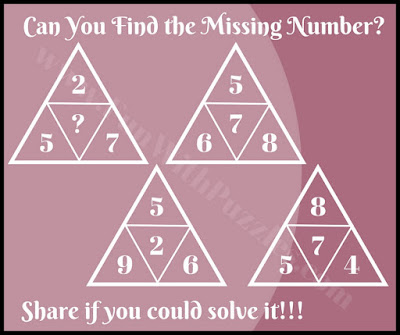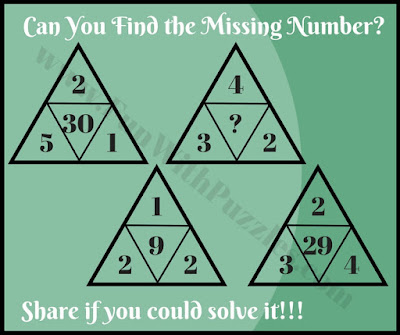Mathematical IQ Riddles are very fun to solve. Earlier we published many Math IQ Riddles in which one has to find the missing number. In most of these math brain Teasers, one is given a set of few numbers which are related to each other or follow some sequence. Challenge is to find the relationship between these given numbers and then solve it for the missing number which will replace the question mark.
In these Math IQ Riddles, four numbers are given inside a triangle. These four numbers are related to each other. There are four triangles each having four numbers. The same relationship among these four numbers is repeated in each of the triangles. One has to find these relations and then tell the number which is missing in one of the triangles.
So are you ready to take this Math IQ challenge? Most of these IQ Riddles are easy. However, in the end, a couple of these riddles are comparatively tough which may shake your brain. The answer to these puzzles is given at the end of this post. Do post your answers in the comment section.1. Easy math triangle IQ riddle2. Fun math triangle IQ riddle3. Simple math triangle IQ riddle4. Mind breaking math triangle IQ riddle5. Tough math IQ riddle triangle picture Puzzle

Unknown said...

29

Rajesh Kumar said...

It will be great if you can post the riddle number for which you posted this answer?

Jay said...

Is the answer to #5 also 5?

Rajesh Kumar said...

Our answer to the last puzzle is not 5. However, if you are able to get the answer 5 for the #5th logical puzzle, it will be interesting to know your logical reasoning to get this answer.

Unknown said...

I solve this five problem in just 25 second only any one who can beat me

1)8
2)4
3)4
4)3
5)29

Unknown said...

Ok